Make nice violin plots easily with 95% (possibly bootstrapped) confidence intervals.

## Usage

nice_violin(
data,
response,
group = NULL,
boot = FALSE,
bootstraps = 2000,
colours,
xlabels = NULL,
ytitle = response,
xtitle = NULL,
has.ylabels = TRUE,
has.xlabels = TRUE,
comp1 = 1,
comp2 = 2,
signif_annotation = NULL,
signif_yposition = NULL,
signif_xmin = NULL,
signif_xmax = NULL,
ymin,
ymax,
yby = 1,
CIcap.width = 0.1,
obs = FALSE,
alpha = 1,
border.colour = "black",
border.size = 2,
has.d = FALSE,
d.x = mean(c(comp1, comp2)) * 1.1,
d.y = mean(data[[response]]) * 1.3,
groups.order = "none",
xlabels.angle = 0
)

## Arguments

data

The data frame.

response

The dependent variable to be plotted.

group

The group by which to plot the variable.

boot

Logical, whether to use bootstrapping for the confidence interval or not.

bootstraps

How many bootstraps to use.

colours

Desired colours for the plot, if desired.

xlabels

The individual group labels on the x-axis.

ytitle

An optional y-axis label, if desired.

xtitle

An optional x-axis label, if desired.

has.ylabels

Logical, whether the x-axis should have labels or not.

has.xlabels

Logical, whether the y-axis should have labels or not.

comp1

The first unit of a pairwise comparison, if the goal is to compare two groups. Automatically displays *, **, or *** depending on significance of the difference. Can take either a numeric value (based on the group number) or the name of the group directly. Must be provided along with argument comp2.

comp2

The second unit of a pairwise comparison, if the goal is to compare two groups. Automatically displays "", "", or "" depending on significance of the difference. Can take either a numeric value (based on the group number) or the name of the group directly. Must be provided along with argument comp1.

signif_annotation

Manually provide the required annotations/numbers of stars (as character strings). Useful if the automatic pairwise comparison annotation does not work as expected, or yet if one wants more than one pairwise comparison. Must be provided along with arguments signif_yposition, signif_xmin, and signif_xmax.

signif_yposition

Manually provide the vertical position of the annotations/stars, based on the y-scale.

signif_xmin

Manually provide the first part of the horizontal position of the annotations/stars (start of the left-sided bracket), based on the x-scale.

signif_xmax

Manually provide the second part of the horizontal position of the annotations/stars (end of the right-sided bracket), based on the x-scale.

ymin

The minimum score on the y-axis scale.

ymax

The maximum score on the y-axis scale.

yby

How much to increase on each "tick" on the y-axis scale.

CIcap.width

The width of the confidence interval cap.

obs

Logical, whether to plot individual observations or not. The type of plotting can also be specified, either "dotplot" (same as obs = TRUE for backward compatibility) or "jitter", useful when there are a lot of observations.

alpha

The transparency of the plot.

border.colour

The colour of the violins border.

border.size

The size of the violins border.

has.d

Whether to display the d-value.

d.x

The x-axis coordinates for the d-value.

d.y

The y-axis coordinates for the d-value.

groups.order

How to order the group factor levels on the x-axis. Either "increasing" or "decreasing", to order based on the value of the variable on the y axis, or "string.length", to order from the shortest to the longest string (useful when working with long string names). "Defaults to "none".

xlabels.angle

How much to tilt the labels of the x-axis. Useful when working with long string names. "Defaults to 0.

## Value

A violin plot of class ggplot, by group.

## Details

Using boot = TRUE uses bootstrapping (for the confidence intervals only) with the BCa method, using the rcompanion_groupwiseMean function.

For the easystats equivalent, see: see::geom_violindot().

Visualize group differences via scatter plots: nice_scatter. Tutorial: https://rempsyc.remi-theriault.com/articles/violin

## Examples

# Make the basic plot
nice_violin(
data = ToothGrowth,
response = "len"
)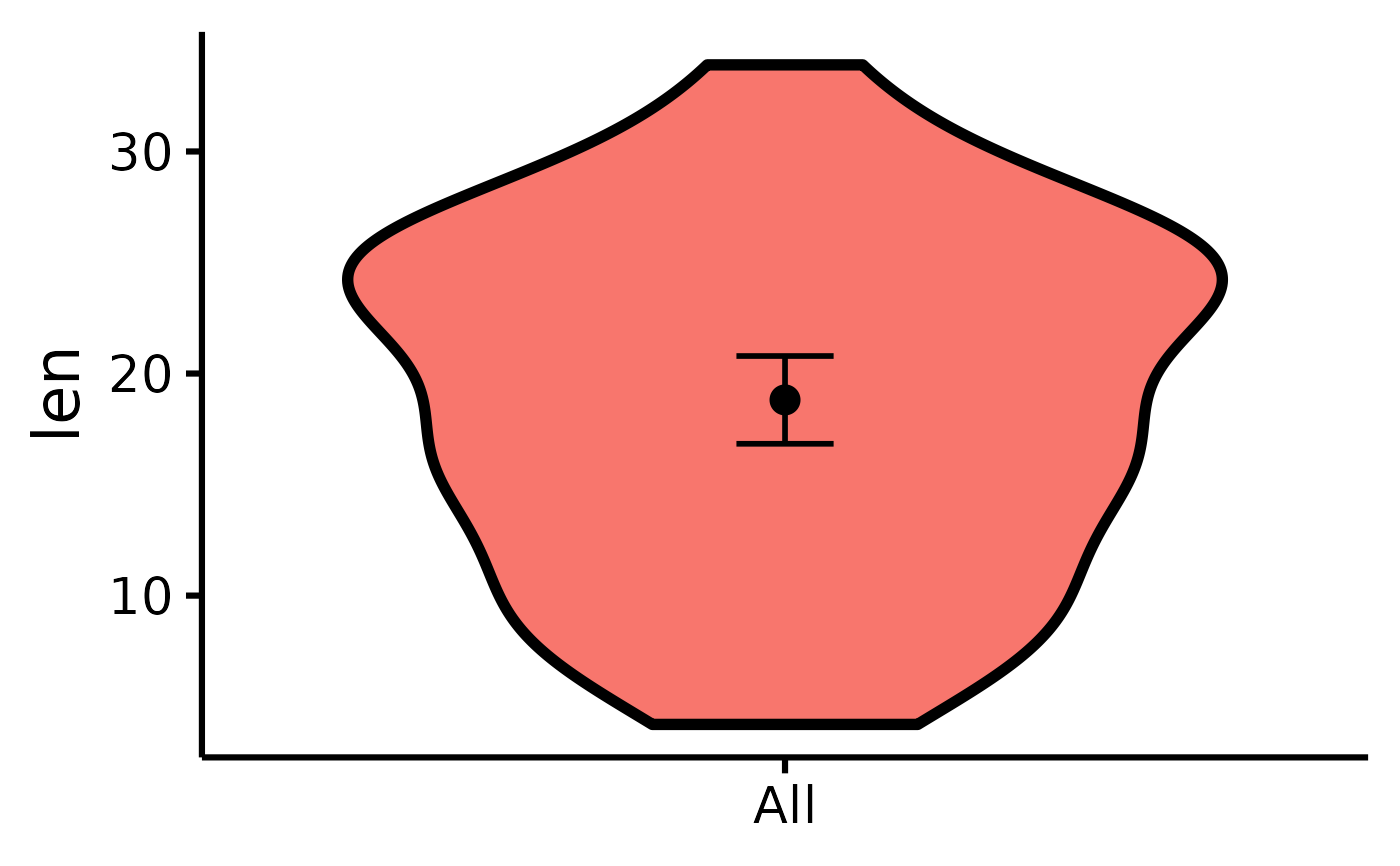# \donttest{
.old_wd <- setwd(tempdir())
# Save a high-resolution image file to specified directory
ggplot2::ggsave("niceviolinplothere.pdf", width = 7,
height = 7, unit = "in", dpi = 300
) # change for your own desired path
setwd(.old_wd)
# Change x- and y- axes labels
nice_violin(
data = ToothGrowth,
group = "dose",
response = "len",
ytitle = "Length of Tooth",
xtitle = "Vitamin C Dosage"
)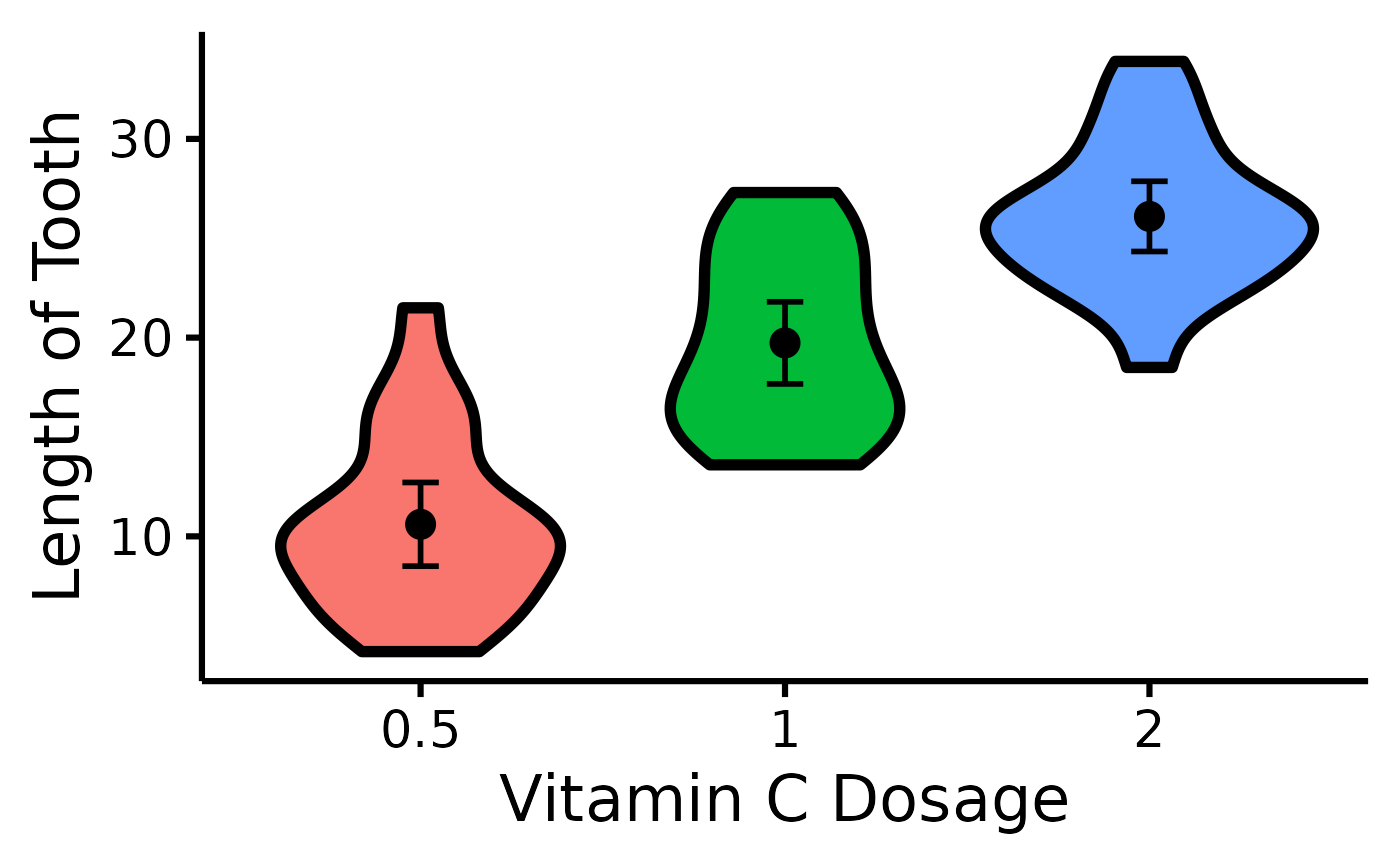# See difference between two groups
nice_violin(
data = ToothGrowth,
group = "dose",
response = "len",
comp1 = "0.5",
comp2 = "2"
)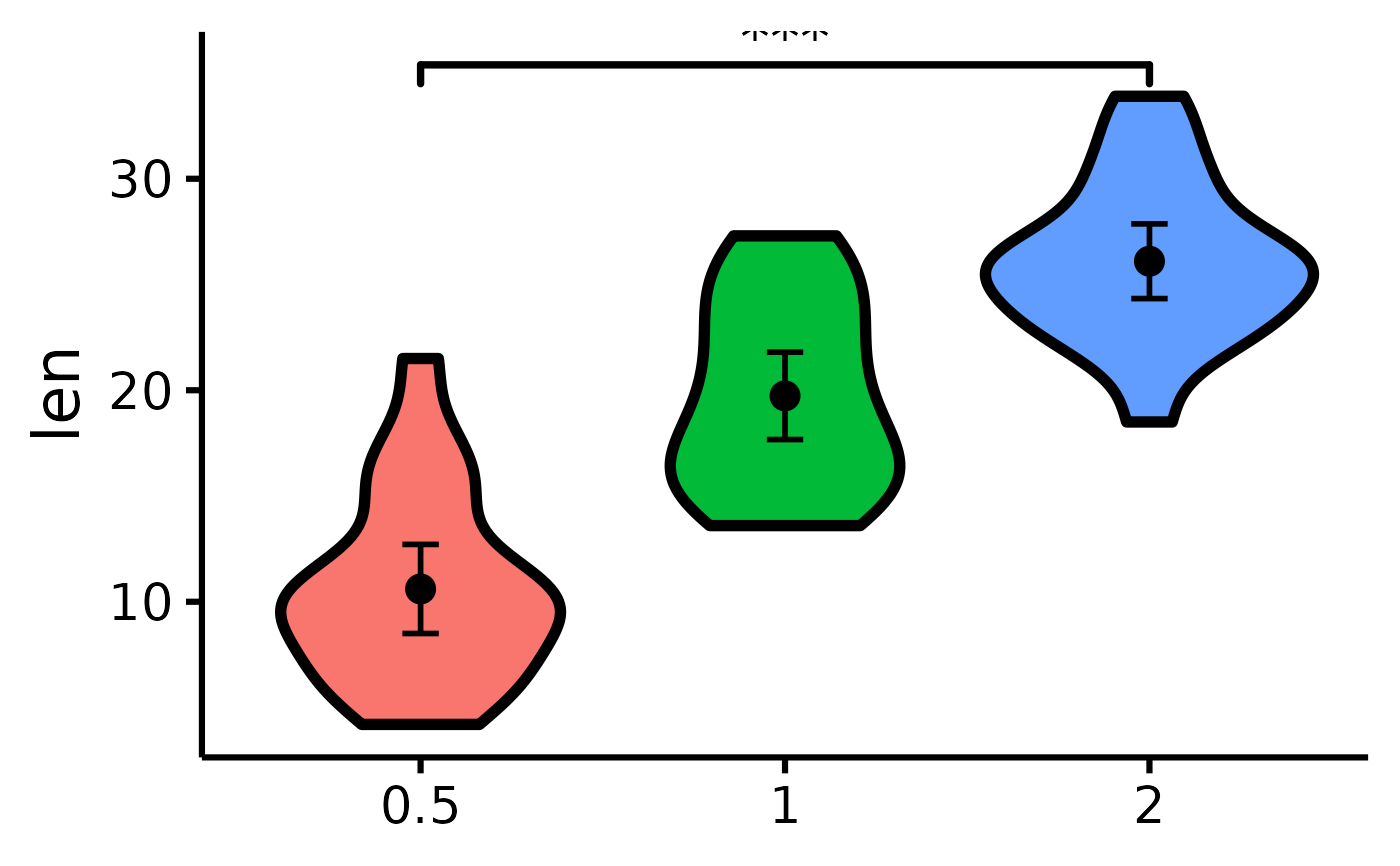nice_violin(
data = ToothGrowth,
group = "dose",
response = "len",
comp1 = 2,
comp2 = 3
)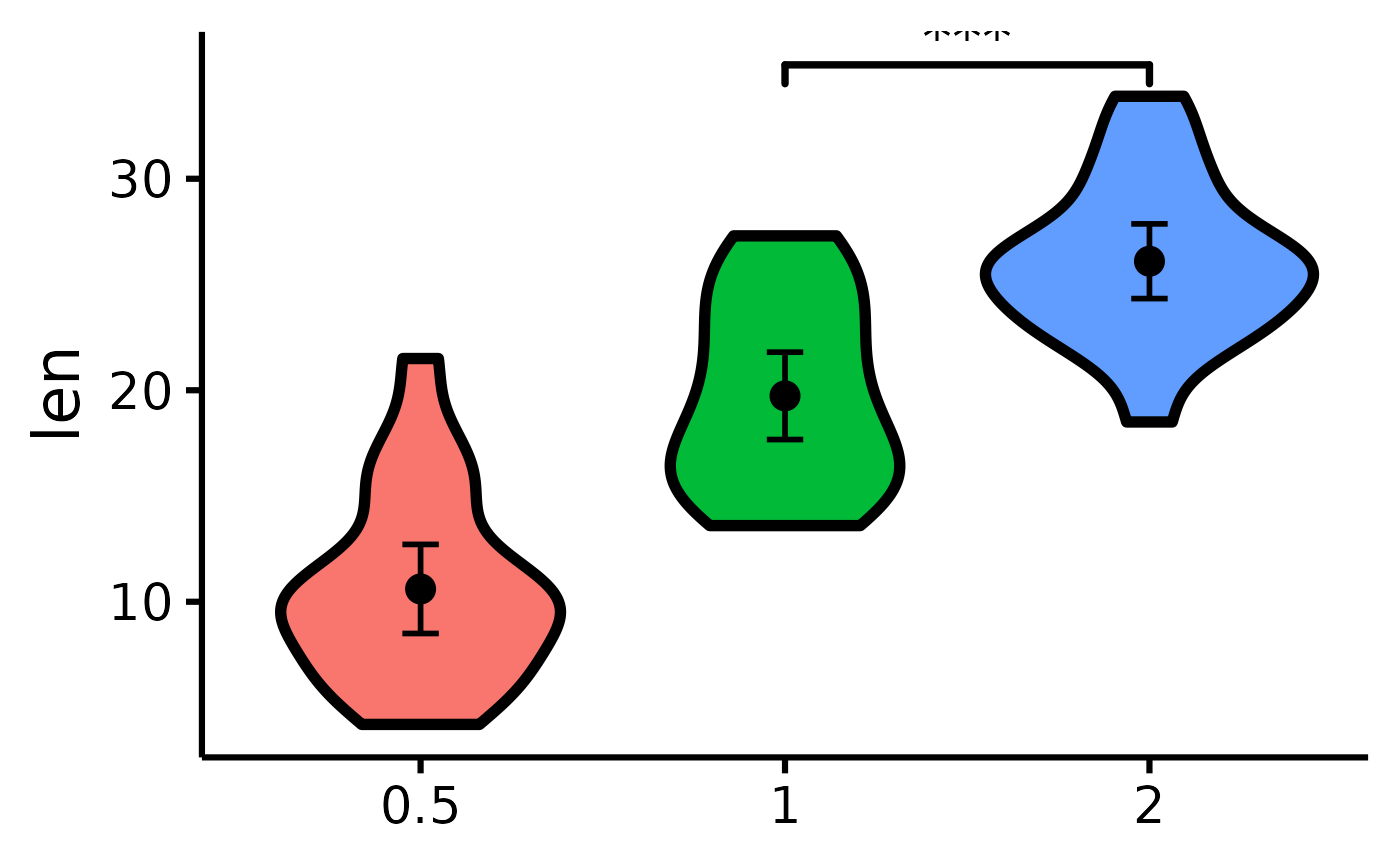# Compare all three groups
nice_violin(
data = ToothGrowth,
group = "dose",
response = "len",
signif_annotation = c("*", "**", "***"),
# manually enter the number of stars
signif_yposition = c(30, 35, 40),
# What height (y) should the stars appear
signif_xmin = c(1, 2, 1),
# Where should the left-sided brackets start (x)
signif_xmax = c(2, 3, 3)
)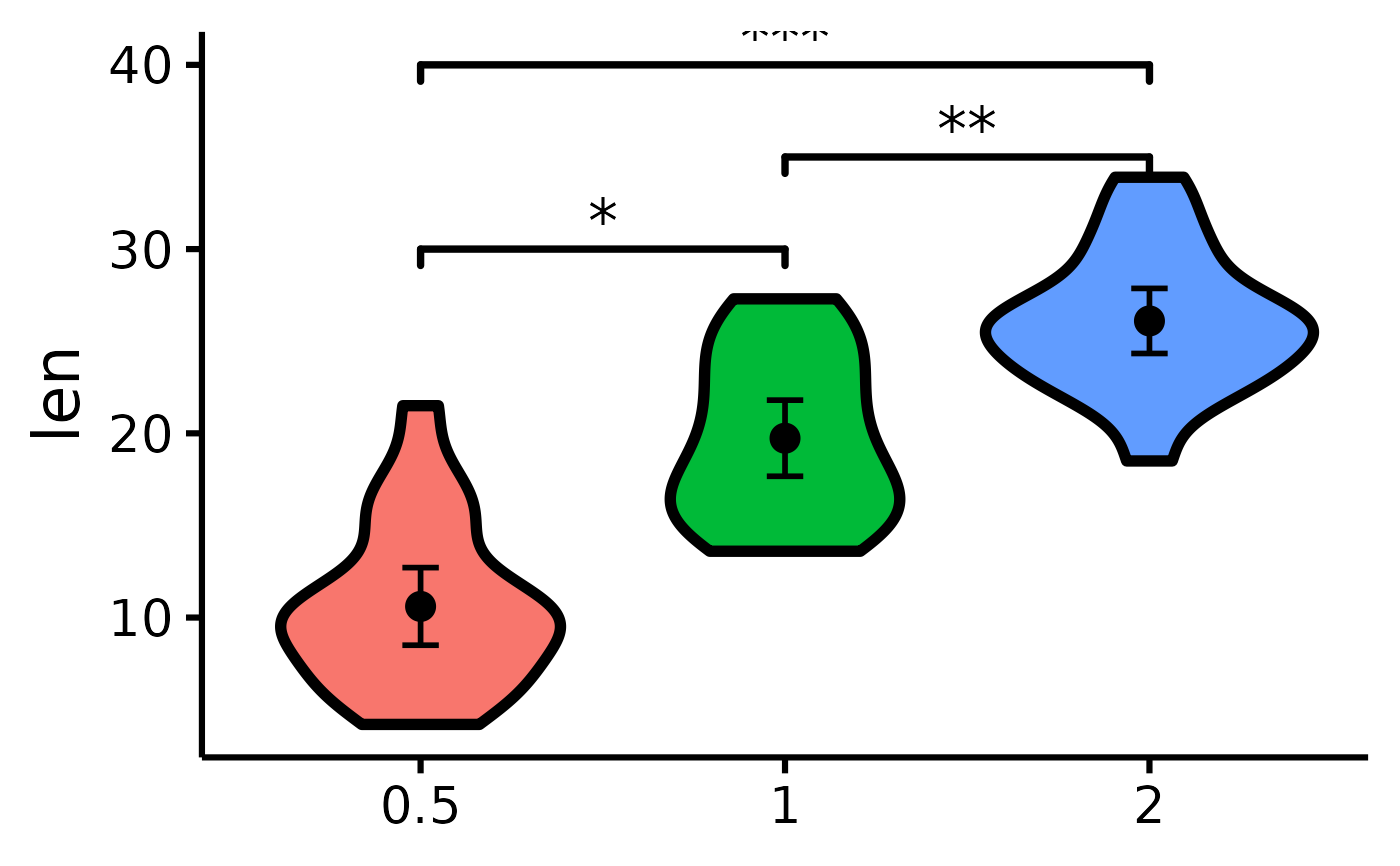# Where should the right-sided brackets end (x)

# Set the colours manually
nice_violin(
data = ToothGrowth,
group = "dose",
response = "len",
)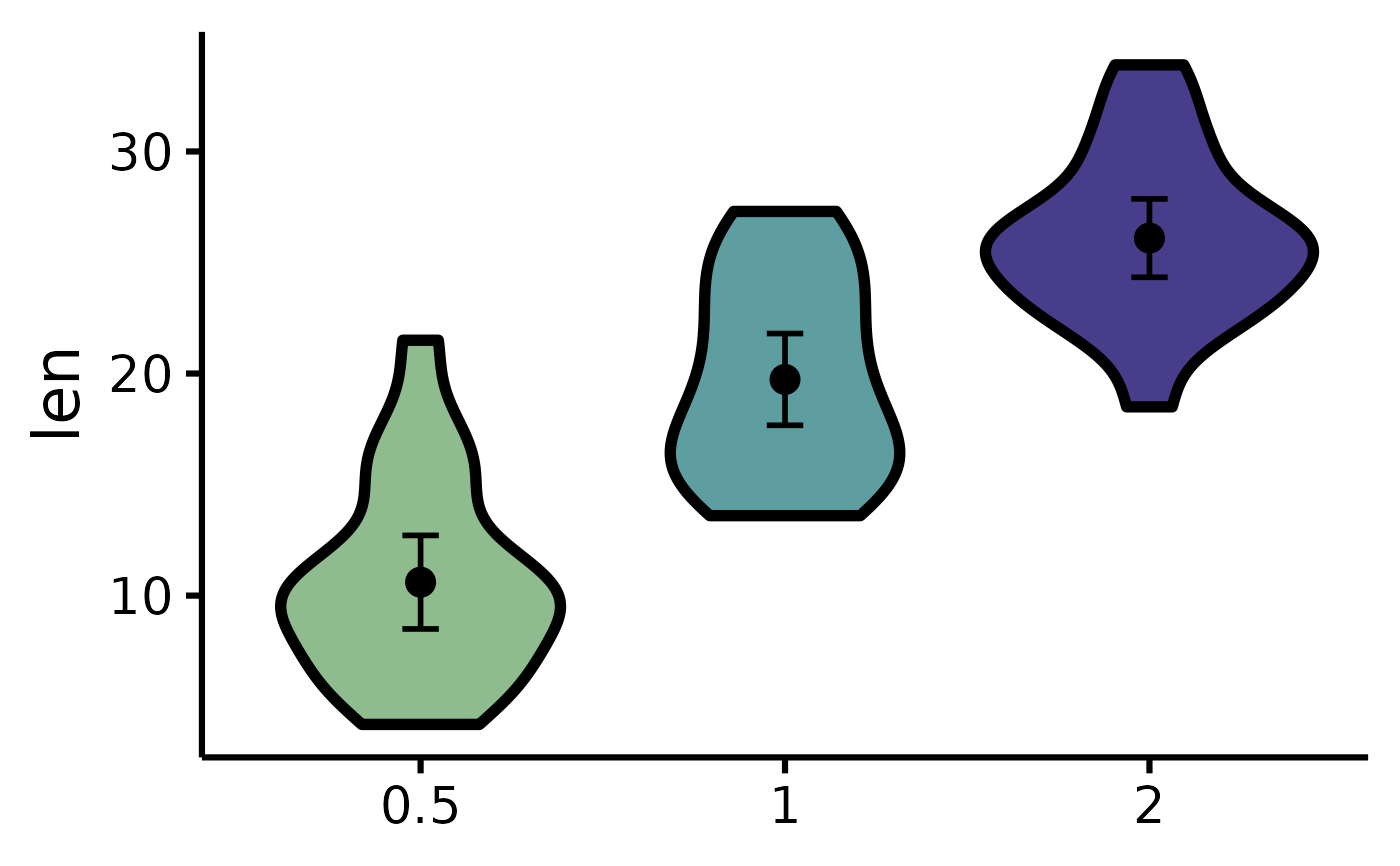# Changing the names of the x-axis labels
nice_violin(
data = ToothGrowth,
group = "dose",
response = "len",
xlabels = c("Low", "Medium", "High")
)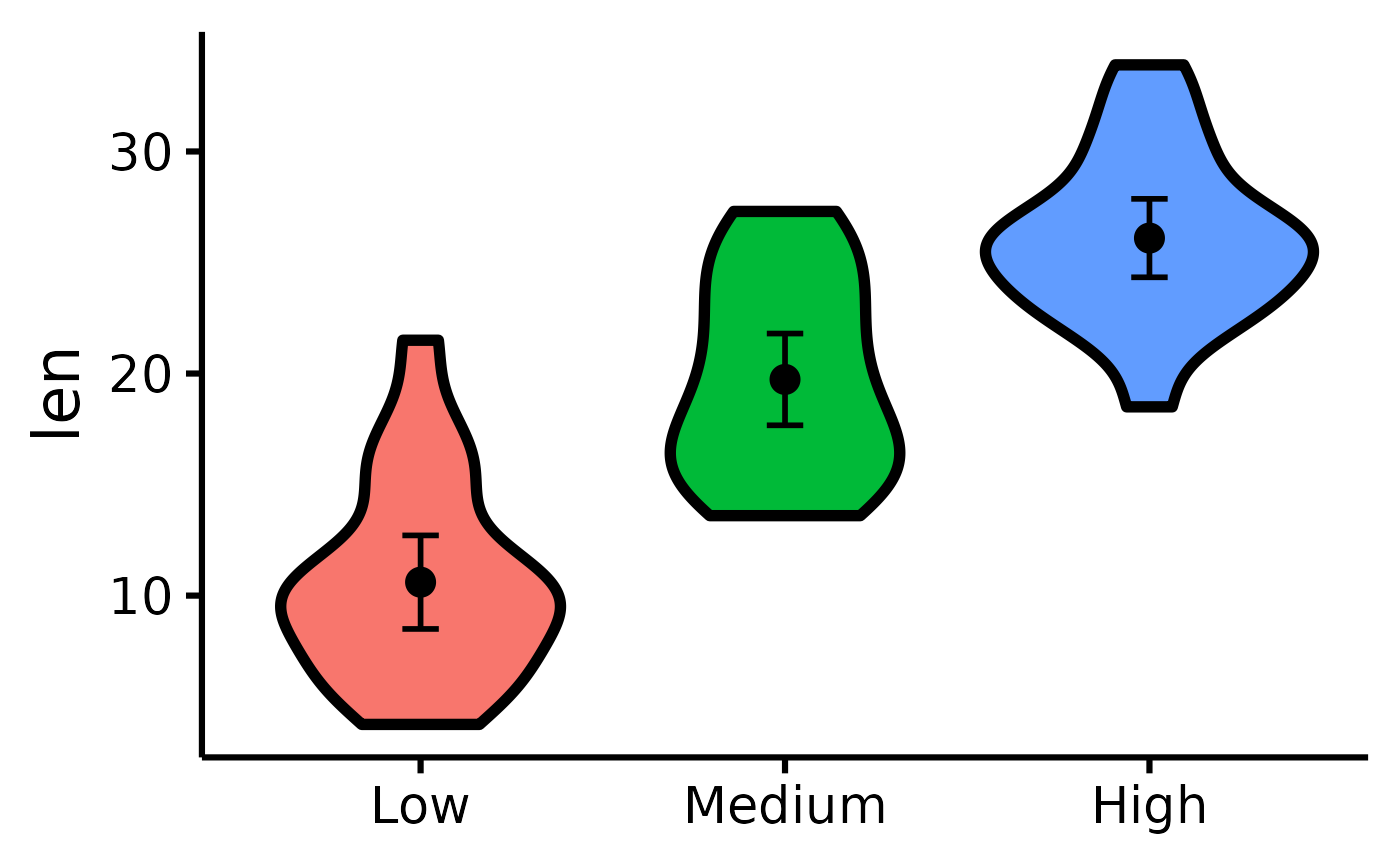# Removing the x-axis or y-axis titles
nice_violin(
data = ToothGrowth,
group = "dose",
response = "len",
ytitle = NULL,
xtitle = NULL
)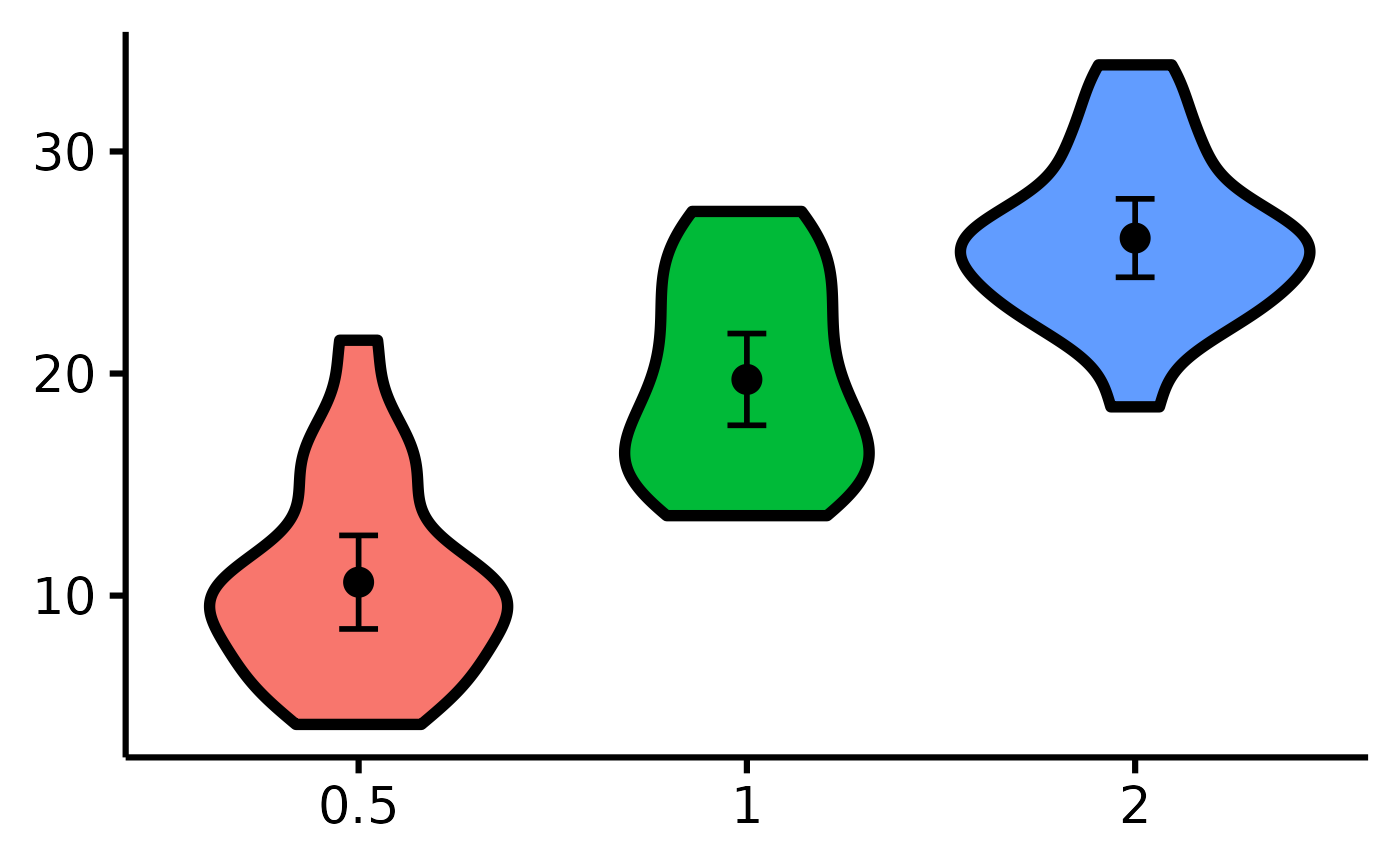# Removing the x-axis or y-axis labels (for whatever purpose)
nice_violin(
data = ToothGrowth,
group = "dose",
response = "len",
has.ylabels = FALSE,
has.xlabels = FALSE
)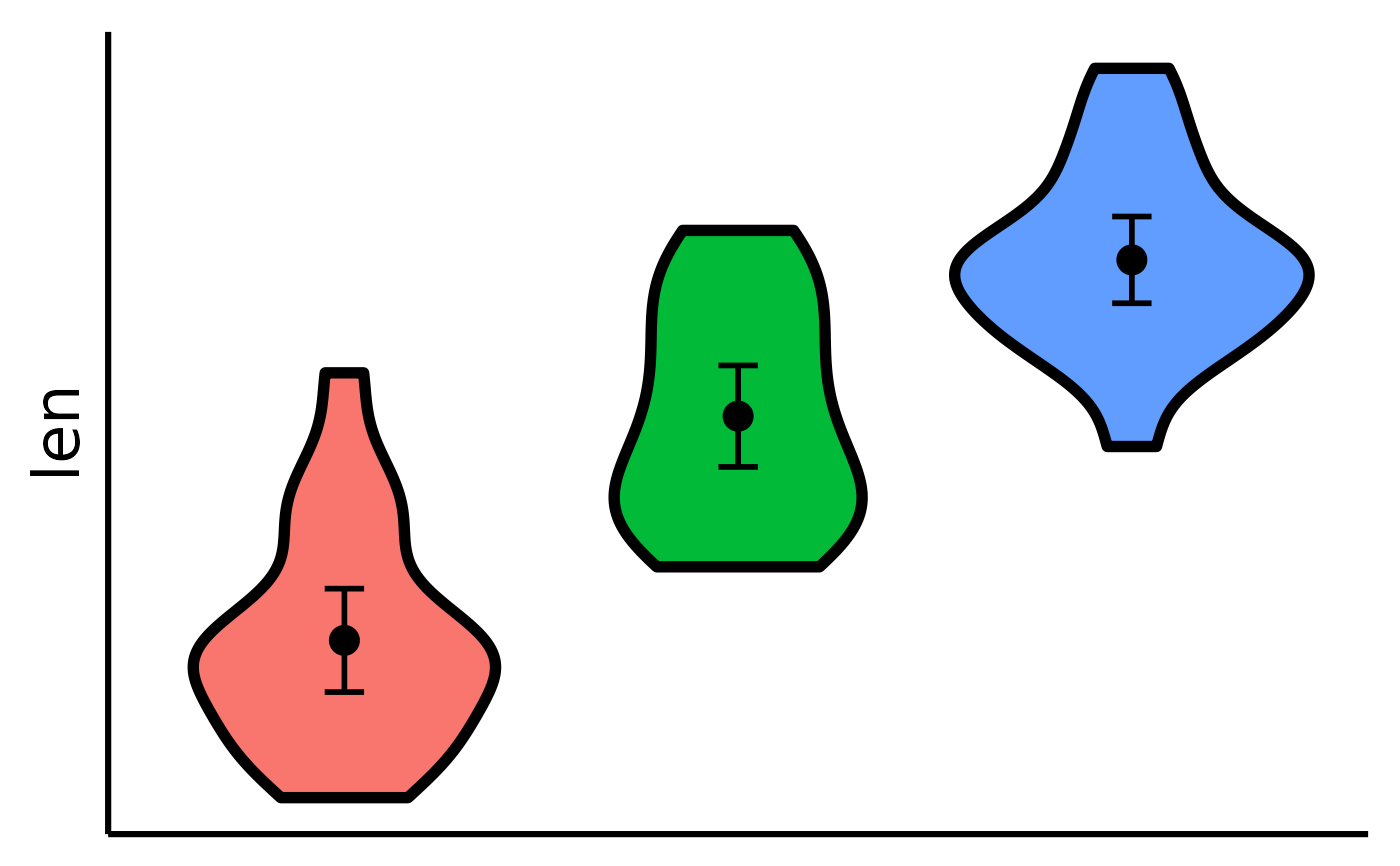# Set y-scale manually
nice_violin(
data = ToothGrowth,
group = "dose",
response = "len",
ymin = 5,
ymax = 35,
yby = 5
)
#> Warning: Removed 1 rows containing non-finite values (stat_ydensity()).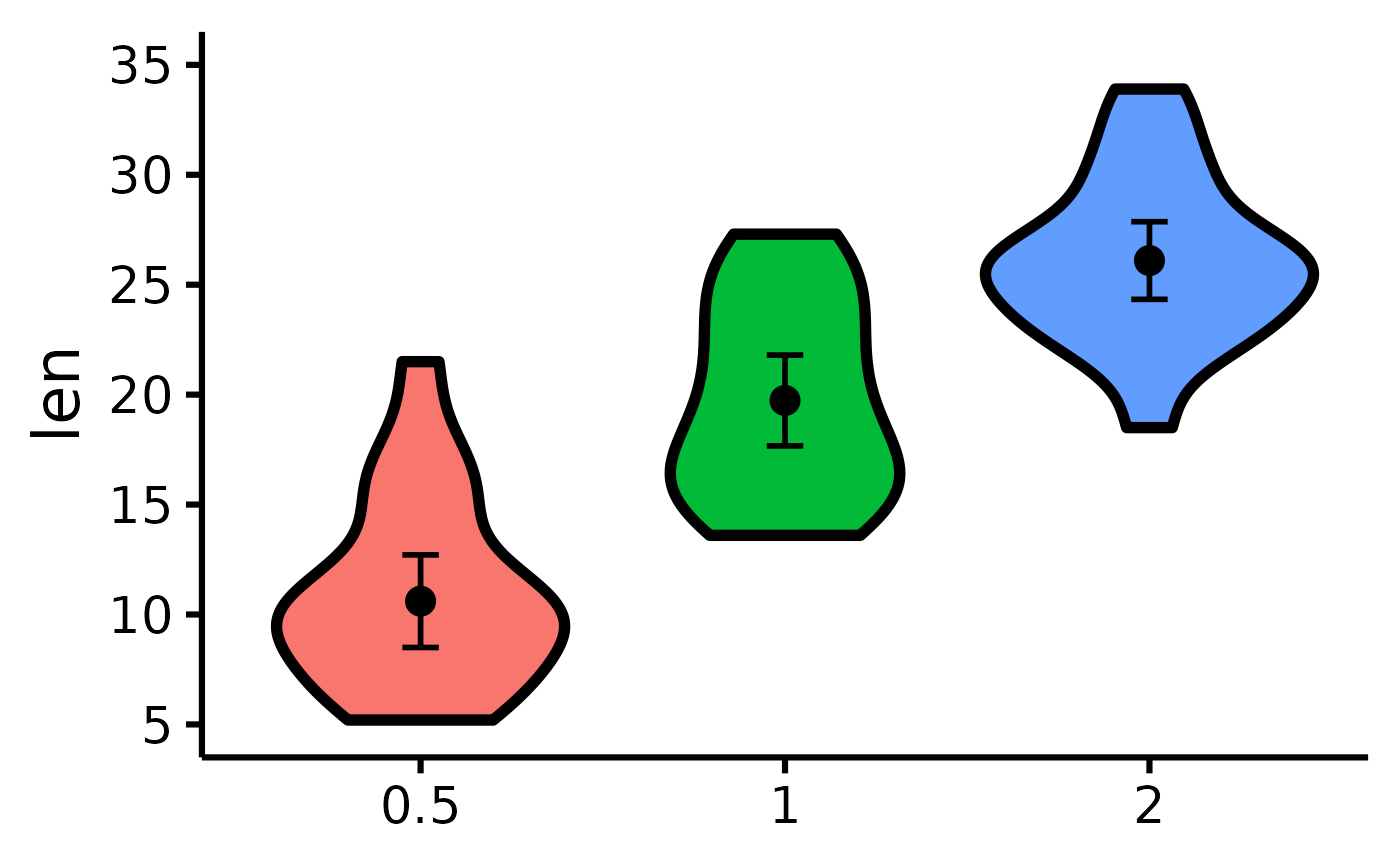# Plotting individual observations
nice_violin(
data = ToothGrowth,
group = "dose",
response = "len",
obs = TRUE
)
#> Bin width defaults to 1/30 of the range of the data. Pick better value with
#> binwidth.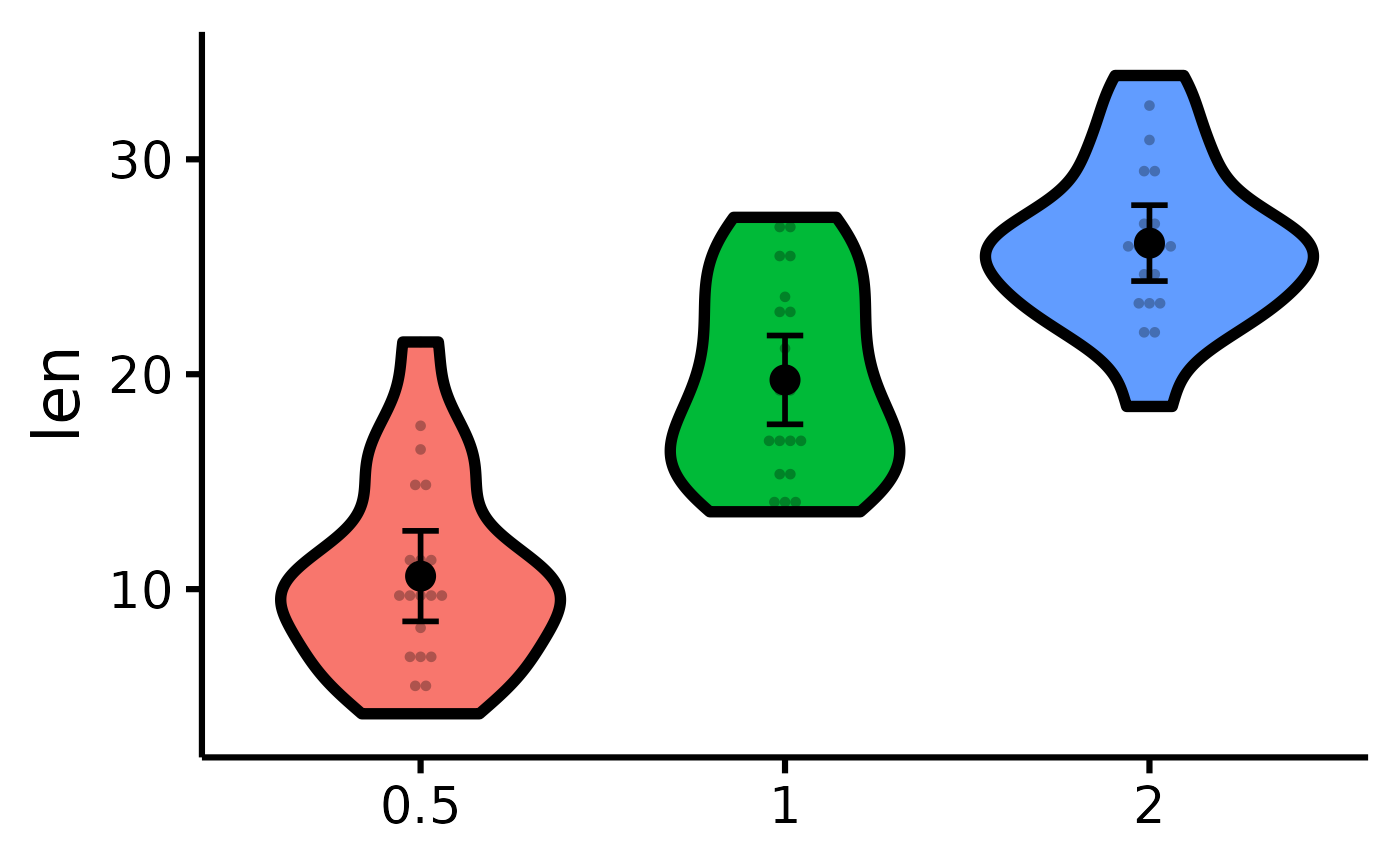# Micro-customizations
nice_violin(
data = ToothGrowth,
group = "dose",
response = "len",
CIcap.width = 0,
alpha = .70,
border.size = 1,
border.colour = "white",
comp1 = 1,
comp2 = 2,
has.d = TRUE
)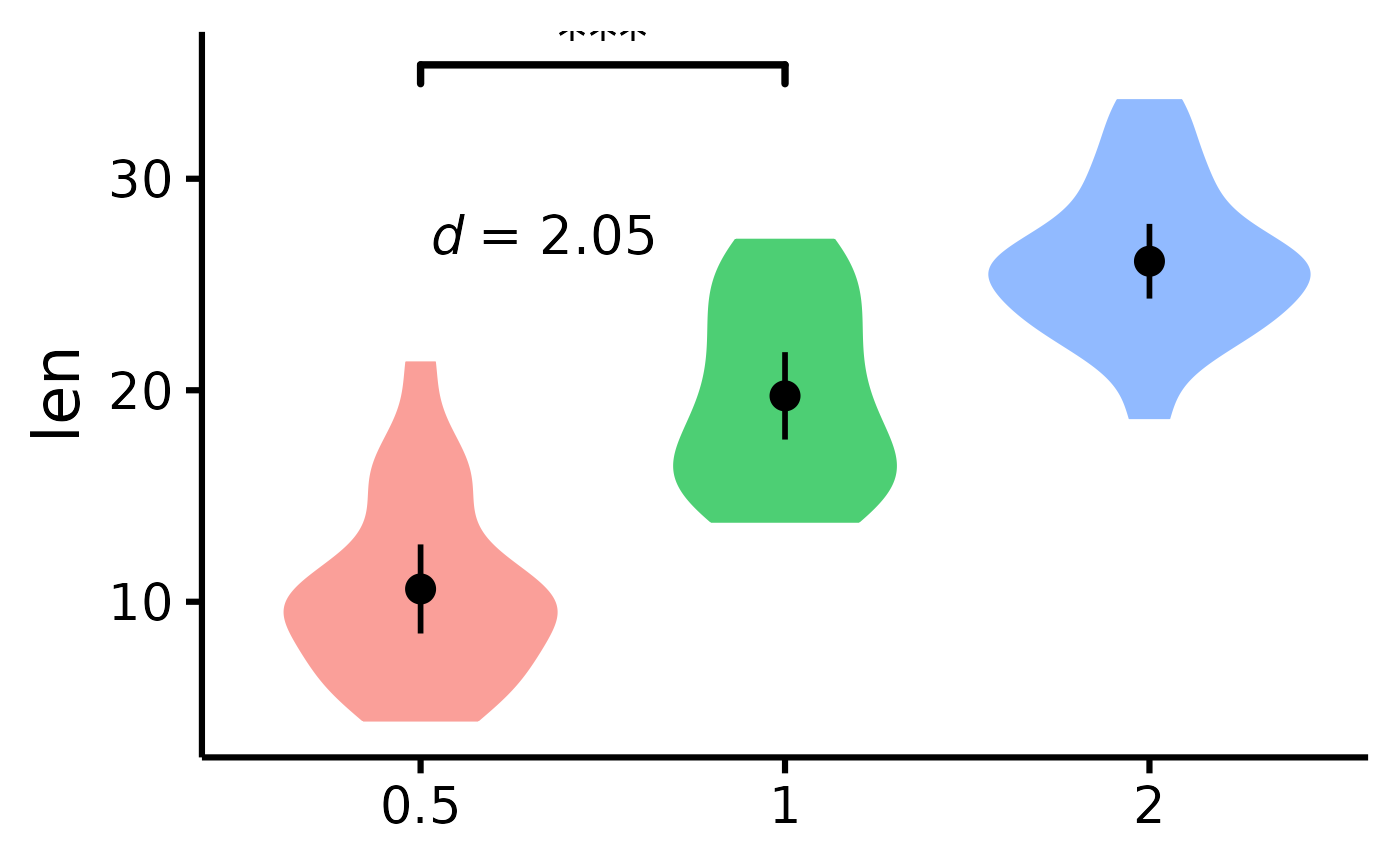# }